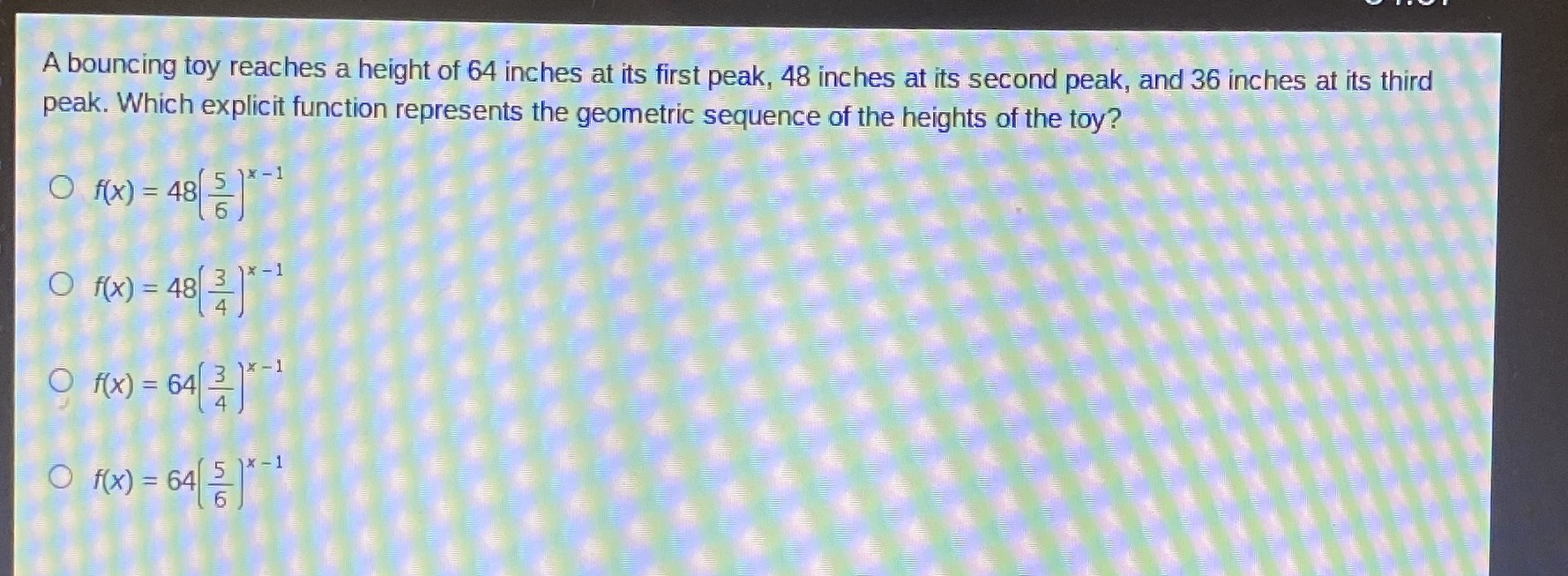### ¿Todavía tienes preguntas de matemáticas?

Pregunte a nuestros tutores expertos
Algebra
PreguntaA bouncing toy reaches a height of $$64$$ inches at its first peak, $$48$$ inches at its second peak, and $$36$$ inches at its third peak. Which explicit function represents the geometric sequence of the heights of the toy?

$$f ( x ) = 48 ( \frac { 5 } { 6 } ) ^ { x - 1 }$$

$$f ( x ) = 48 ( \frac { 3 } { 4 } ) ^ { x - 1 }$$

$$f ( x ) = 64 ( \frac { 3 } { 4 } ) ^ { x - 1 }$$

$$f ( x ) = 64 ( \frac { 5 } { 6 } ) ^ { x - 1 }$$

f(x) = 64$$(\frac{3}{4})^{x- 1}$$